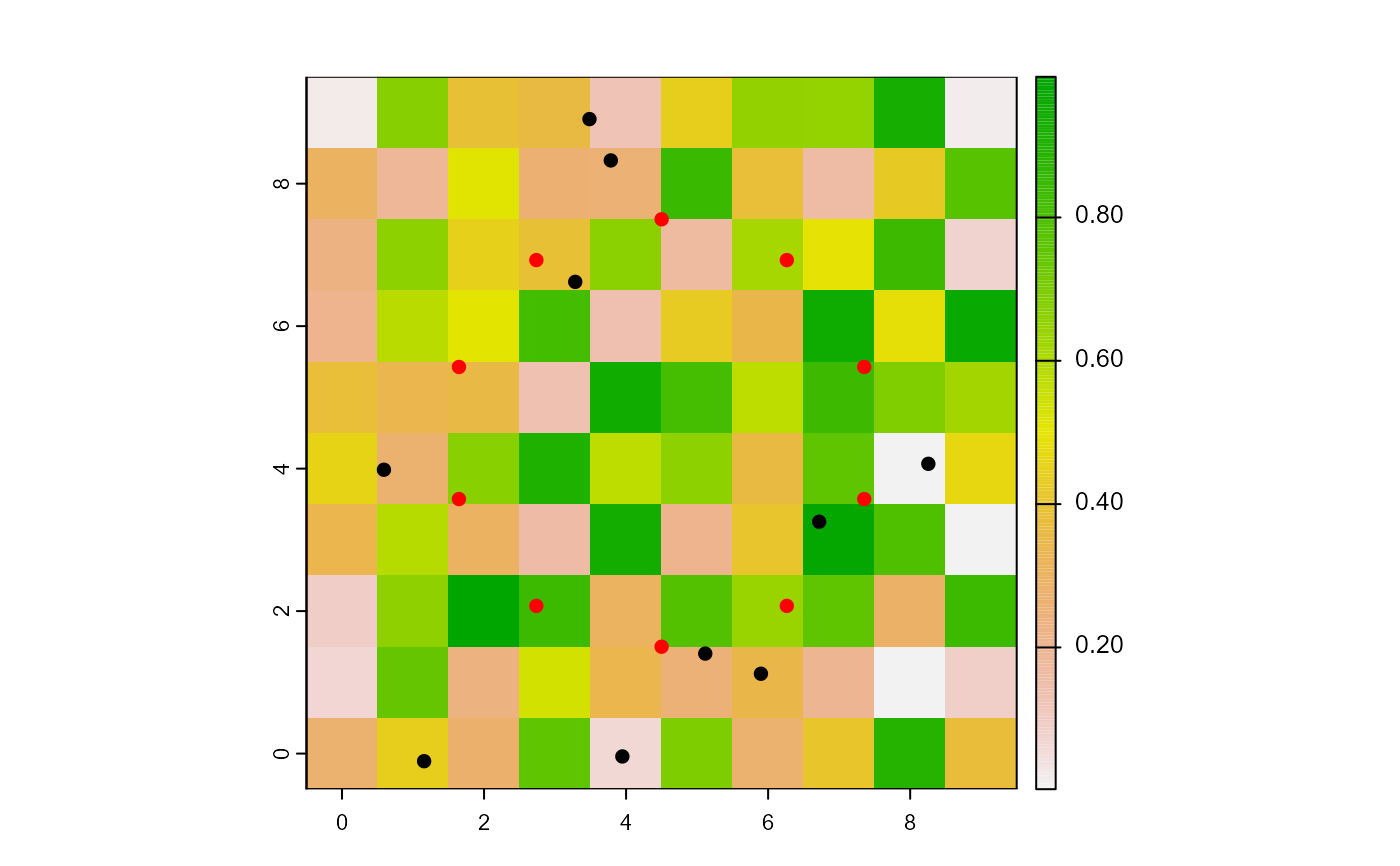Relocate the turtles on a circle centered on the world.

layoutCircle(world, turtles, radius, torus = FALSE)

# S4 method for worldNLR,agentMatrix,numeric
layoutCircle(world, turtles, radius, torus = FALSE)

## Arguments

world

WorldMatrix or worldArray object.

turtles

AgentMatrix object representing the moving agents.

torus

Logical to determine if the world is wrapped. Default is torus = FALSE.

## Value

AgentMatrix representing the turtles with updated coordinates and updated data for their heading values and previous coordinates prevX and prevY.

## Details

The turtles point outwards.

If the radius value leads turtles outside of the world's extent and torus = TRUE, they are relocated on the other sides of the world, inside its extent; if torus = FALSE, the turtles are located past the world's extent.

Sarah Bauduin

## Examples

w1 <- createWorld(minPxcor = 0, maxPxcor = 9, minPycor = 0, maxPycor = 9,
data = runif(100))
t1 <- createTurtles(n = 10, coords = randomXYcor(w1, n = 10))
plot(w1)
points(t1, col = "black", pch = 16)

t1 <- layoutCircle(world = w1, turtles = t1, radius = 3)
points(t1, col = "red", pch = 16)